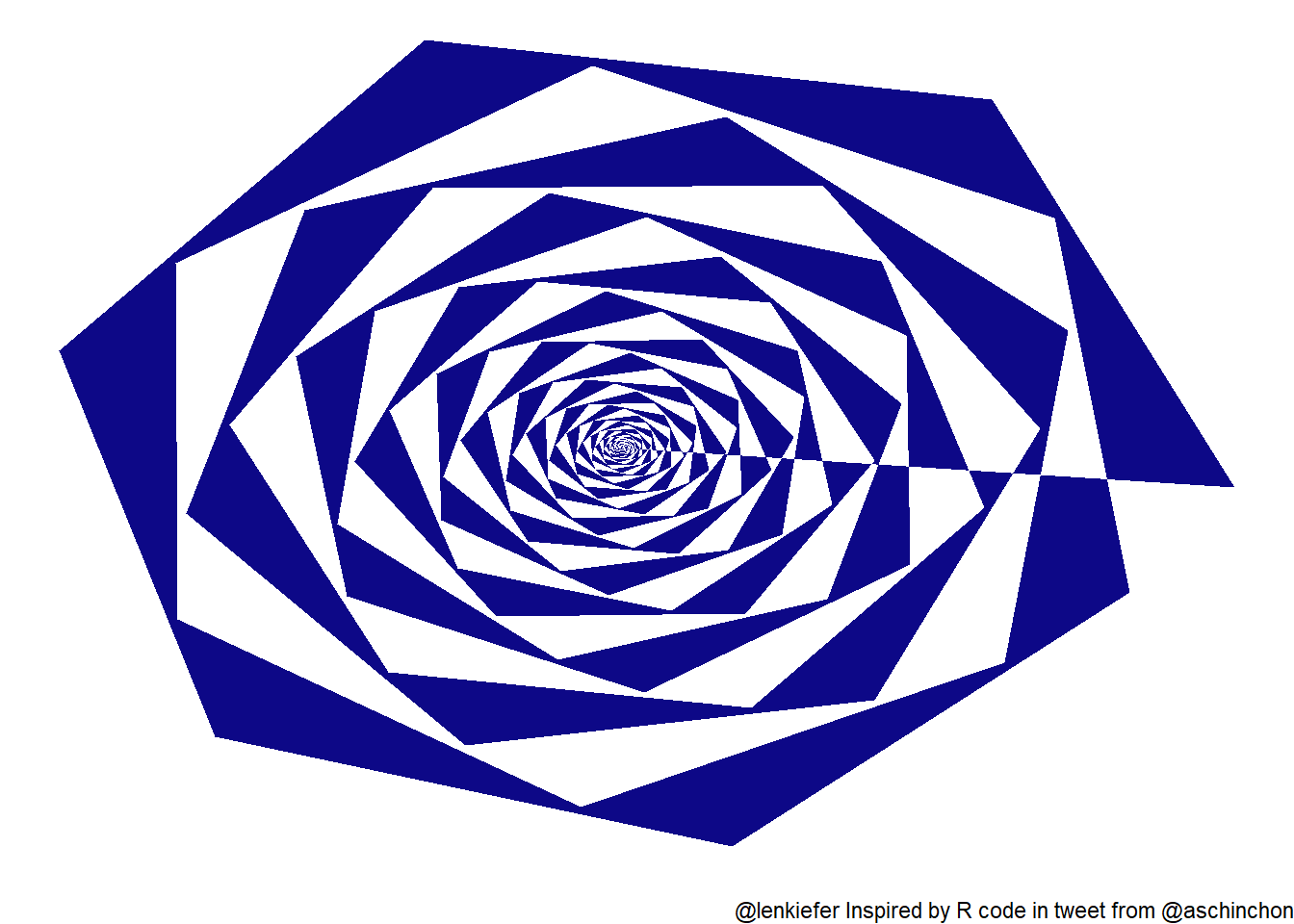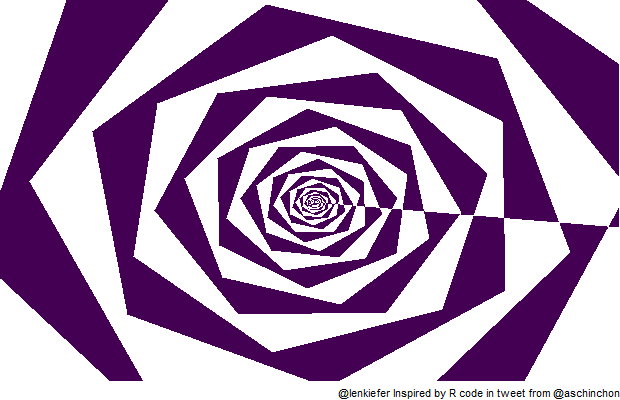# Everything is spiraling out of control!

## Making a fun spiral dataviz with R.

I saw this fun bit of R code in a tweet by user aschinchon.

Let’s remix it to make a fun animation. We’ll zoom in and out and have the colors shift.

Click for R code

suppressPackageStartupMessages({
library(tidyverse)
library(tweenr)
library(gifski)
library(viridis)
})

df <- data.frame(x=0, y=0)

for (i in 2:500)
{
df[i,1] <- df[i-1,1]+((0.98)^i)*cos(i)
df[i,2] <- df[i-1,2]+((0.98)^i)*sin(i)
}

# function to make code
myf <- function(rr, fill="#0D0887FF"){
df <- data.frame(x=0, y=0)

for (i in 2:500){
df[i,1] <- df[i-1,1]+((rr)^i)*cos(i)
df[i,2] <- df[i-1,2]+((rr)^i)*sin(i)
}
df$fill <- fill return(df) } # plot g<- ggplot(myf(0.98), aes(x,y,fill=fill)) + geom_polygon()+ scale_fill_identity()+ theme_void()+ labs(caption="@lenkiefer Inspired by R code in tweet from @aschinchon") g# Animate it We can animate it with gifski and tweenr with the following code. Click for R code # change this # Set to your directory mydir<-"YOUR_DIRECTORY" # Create a set of data frames to tween mylist<-list(myf(.970, viridis(8)), myf(.975, viridis(8)), myf(.980, viridis(8)), myf(.985, viridis(8)), myf(.990, viridis(8)), myf(.985, viridis(8)), myf(.980, viridis(8)), myf(.975, viridis(8)), myf(.970, viridis(8)) ) tween.df<-tween_states(mylist,tweenlength=5,statelength=0, ease=rep('cubic-in-out',20), nframes=50) N <- max(tween.df$.frame)

gif_file <- save_gif({for (i in seq(1:N)){
g<-
ggplot(data=filter(tween.df,.frame==i), aes(x,y,fill=fill)) +
geom_polygon()+
scale_fill_identity()+
theme_void()+
coord_cartesian(xlim=c(-1.5,-0.5), ylim=c(-0.25,0.5))+   # zooom in
labs(caption="@lenkiefer Inspired by R code in tweet from @aschinchon")

print(g)
print(paste(i,"out of",N))
}
}, gif_file= paste0(mydir,"/spiral.gif"),width = 620, height = 400, res = 92, delay=1/20)

Running the code yields:You can play with the colors or change the parameters to speed up or slow down this animation. Adding to nframes in tweenr::tween_states can make the animation smoother. And increasing/decreasing delay in gifski::save_gif() can speed it up/slow it down.